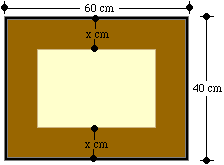Sender: Jennifer Date: Thu, 4 Mar 1999 18:13:11 -0800 To Whom It Concern: I've been having difficulty with this one question involving area. The level of the question is Grade 11/12. Here it is!    A rink is 40 m long by 20 m wide. There are plans to enlarge it by 700m^2 by adding a strip at one end and a strip of the same width along one side. Find the width of the strip. (Let the width of the strip by x meters.) Hi Jennifer The area of the present rink is 20 m times 40 m = 800 sq m so the area of the new rink will be 1500 sq m. Let the width of the strip by x meters. The key to this problem is to draw a sketch, it should look something like this.The enlarged rink is thus (40 + x) m by (20 + x) m and hence its area is (40 + x)(20 + x) sq m. Since the area is 1500 sq m you have that (40 + x)(20 + x) = 1500.    If you expand the left hand side and simplify by collecting like terms you will see that this is a quadratic equation which you can solve by factoring or by using the quadratic equation formula. Since the equation is a quadratic you will probably get two different values for x but only one will make sense. Cheers Jack

Go to Math Central